Next: Life expectancy Up: Methods of calculation of Previous: Methods of calculation of

# A semi-markovian process

Let us call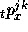the probability, for a person of age x and in status j at time 0, of being in status k at time t.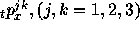can be obtained by solving the ``forward Kolmogorov equations'', which decompose interval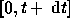into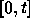and.

Introducing the 4 transition forces: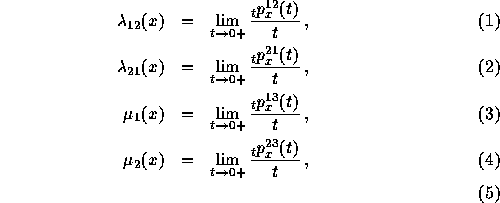one can write for example: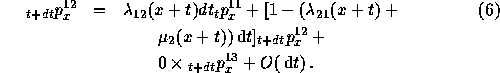These 6 differential equations can also be written (8):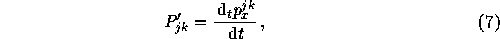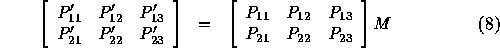with :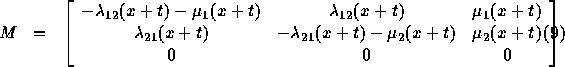Last equations, involving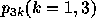have been suppressed for simplicity. Two of the four equations are redundant, because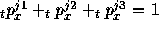. The system can therefore clearly be reduced to a set of two first order differential equations involving four forces and submatrix :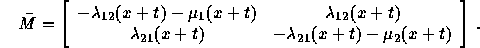Nicolas Brouard
Tue Jun 6 00:15:46 DF 1995